Function Repository Resource:

# RealToHexString

Give a string representing the hexadecimal form of a real number

Contributed by: Daniel Lichtblau
 ResourceFunction["RealToHexString"][n,{e,h}] gives the hexadecimal string corresponding to n, with an exponent field of e bits and a total length of h hex digits. ResourceFunction["RealToHexString"][n] gives the hexadecimal string for n, using the common IEEE double-precision values of 11 exponent bits and 64 bits total.

## Details and Options

ResourceFunction["RealToHexString"] uses rounding on arbitrary-precision inputs in order to get the final digit correct.

## Examples

### Basic Examples (1)

Find the hexadecimal string for the decimal 1.1:

 In:=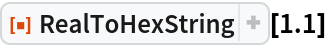Out=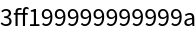### Scope (2)

RealToHexString will handle arbitrary-precision reals:

 In:=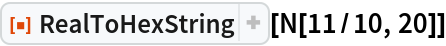Out=RealToHexString can return a string with length exceeding the capacity of a machine double-precision value:

 In:=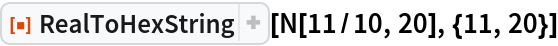Out=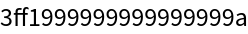### Possible Issues (1)

RealToHexString will pad with zeros if the number of hex digits to return makes it exceed the internal precision of the input:

 In:=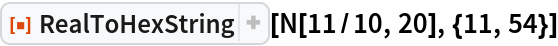Out=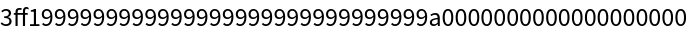## Requirements

Wolfram Language 11.3 (March 2018) or above

## Version History

• 1.0.0 – 28 February 2019